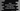# How to remove elements of Java ArrayList using removeIf() method## How to remove elements of Java ArrayList using removeIf() method :

Normally, for removing an element from an arraylist, we iterate through the list one by one using an iterator, check each element if it satisfy a specific condition and if it does, delete it. But, starting from Java-8, we don’t need to write all of these code. Java-8 has introduced one new method removeIt() to remove all elements from a collection that satisfy a condition.

In this example, I will show you how to use removeIt() with examples.

### Syntax of removeIt() :

The syntax of removeIt() as below :

``````public boolean removeIf​(Predicate<? super E> filter)
``````
• The parameter filter is a Predicate. If the given predicate satisfy the condition, the element will be removed.

• This method returns one boolean value : true if elements are removed, false otherwise.

• If the parameter filter is null, it will throw one NullPointerException.

### Example of removeIf() :

Following example will remove all numbers greater than 0 and less than 10 from an arraylist.

``````import java.util.*;

class Example {
public static void main(String args[]) {
//1
ArrayList numList = new ArrayList();

//2

//3
System.out.println("Original list : ");

for (int i : numList) {
System.out.print(i + " ");
}

//4
numList.removeIf(i -> (i > 0 && i < 10));

//5
System.out.println("\nModified list : ");

for (int i : numList) {
System.out.print(i + " ");
}

}
}
``````

### Explanation :

The commented numbers in the above example denote the step number below :

1. Create one ArrayList to hold integer variables.

2. Add different numbers to this list. Add both one digit and two digit numbers.

3. Print out the original arraylist values to the user.

4. Using removeIf method, remove all numbers those are less than 10 and greater than 0 from the arraylist.

5. Print out the modified list again to the user.

### Output :

``````Original list :
41 4 1 2 21 11
Modified list :
41 21 11
``````

Let me show you one more example with custom objects :

``````import java.util.*;

class Student{
String name;
int marks;

public Student(String name, int marks){
this.name = name;
this.marks = marks;
}
}

class Example {
public static void main(String args[]) {

ArrayList studentList = new ArrayList();

studentList.removeIf(student -> (student.marks < 30));

System.out.println("Final list : ");

for (Student student : studentList) {
System.out.println(student.name);
}

}
}
``````

In this program, we have removed all Student objects with marks less than 30. Output :

``````Final list :
Alex
Bob
``````

### Conclusion :

As we have seen in this tutorial that removeIf() method really comes in handy if we are removing elements from a collection in Java. We have also learn how to remove custom object elements using removeIf(). Go through the examples we have explained above and drop one comment below if you have any queries.